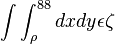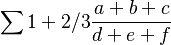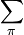# Difference between revisions of "Sandpit"

new math test$\int \int_{\rho}^{88} dx dy \epsilon \zeta$

test math test preview$\sum 1+2/3 \frac{a+b+c}{d+e+f}$

version 1.30.0 test on new

version 1.29 edit test$\sum_{\pi}$

\begin{equation} a = mx +c \int \end{equation}

add + 1 +2 +3 subtract 5-3245